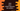# Java program to print hollow diamond star pattern## Java program to print hollow diamond star pattern:

The diamond pattern is similar to printing a hollow triangle pattern. We have to print one hollow triangle and one reverse triangle to print the hollow diamond pattern.

For example, if we have to print the below pattern:

``````     *
* *
*   *
*     *
*       *
*         *
*       *
*     *
*   *
* *
*``````

we will print the upper triangle:

``````     *
* *
*   *
*     *
*       *
*         *``````

and the lower triangle:

`````` *       *
*     *
*   *
* *
*``````

We will break the algorithm into two parts: to print the upper triangle and to print the lower triangle. The program will join these parts to print the complete program.

### Algorithm to print the upper triangle:

We have to use one loop and two inner loops to print the triangles. To print the upper triangle,

• Run one loop from i = 1 to i = height, where height is the height for the upper triangle.
• Run one inner loop from 1 to height - i to print the blank spaces.
• Run another inner loop from 1 to 2*i - 1. For the first and the last iteration, print a star, else print a blank space.
• At the end of each iteration of the main loop, print a new-line character to move to the next line.

### Algorithm to print the lower triangle:

To print the lower triangle, we will have to use a similar algorithm.

• The outer loop will run from i = height - 1 to i > 0 and on each step, the value of i is decremented by 1.
• The inner loops will work in a similar way.

Let’s use these algorithms to print the complete pattern.

### Example 1: Java program to print a hollow diamond pattern:

The below program prints a hollow diamond pattern. It uses the above algorithm:

``````import java.util.Scanner;

public class Main {

public static void main(String[] args) {
Scanner scanner = new Scanner(System.in);

System.out.println("Enter the height of the pattern: ");
int height = scanner.nextInt();

for (int i = 1; i <= height; i++) {
for (int j = 1; j <= height - i; j++) {
System.out.print(" ");
}
for (int k = 1; k <= 2 * i - 1; k++) {
if (k == 1 || k == 2 * i - 1) {
System.out.print("*");
} else {
System.out.print(" ");
}
}
System.out.println();
}

for (int i = height - 1; i > 0; i--) {
for (int j = 1; j <= height - i; j++) {
System.out.print(" ");
}
for (int k = 1; k <= 2 * i - 1; k++) {
if (k == 1 || k == 2 * i - 1) {
System.out.print("*");
} else {
System.out.print(" ");
}
}
System.out.println();
}
}
}``````

It reads the height of the pattern and assigns it to the height variable. If you run this program, it will print outputs as below:

``````Enter the height of the pattern:
5
*
* *
*   *
*     *
*       *
*     *
*   *
* *
*``````

### Example 2: Java program to print a hollow diamond pattern by using a separate method:

We can write a different method and move the logical part to this method. This method will take the height as a parameter and print the pattern.

``````import java.util.Scanner;

public class Main {

private static void printHollowDiamond(int height) {
for (int i = 1; i <= height; i++) {
for (int j = 1; j <= height - i; j++) {
System.out.print(" ");
}
for (int k = 1; k <= 2 * i - 1; k++) {
if (k == 1 || k == 2 * i - 1) {
System.out.print("*");
} else {
System.out.print(" ");
}
}
System.out.println();
}

for (int i = height - 1; i > 0; i--) {
for (int j = 1; j <= height - i; j++) {
System.out.print(" ");
}
for (int k = 1; k <= 2 * i - 1; k++) {
if (k == 1 || k == 2 * i - 1) {
System.out.print("*");
} else {
System.out.print(" ");
}
}
System.out.println();
}
}

public static void main(String[] args) {
Scanner scanner = new Scanner(System.in);

System.out.println("Enter the height of the pattern: ");
int height = scanner.nextInt();

printHollowDiamond(height);
}
}``````

Here, printHollowDiamond is the new method we are using to print the pattern.

You can see that the code in the for loop is similar for both loops. We can move that part to a separate method:

``````import java.util.Scanner;

public class Main {

private static void printPattern(int height, int i) {
for (int j = 1; j <= height - i; j++) {
System.out.print(" ");
}
for (int k = 1; k <= 2 * i - 1; k++) {
if (k == 1 || k == 2 * i - 1) {
System.out.print("*");
} else {
System.out.print(" ");
}
}
System.out.println();
}

private static void printHollowDiamond(int height) {
for (int i = 1; i <= height; i++) {
printPattern(height, i);
}

for (int i = height - 1; i > 0; i--) {
printPattern(height, i);
}
}

public static void main(String[] args) {
Scanner scanner = new Scanner(System.in);

System.out.println("Enter the height of the pattern: ");
int height = scanner.nextInt();

printHollowDiamond(height);
}
}``````

It will make the code clean and concise.